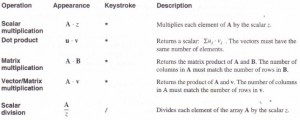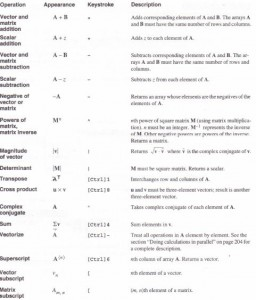# Vector and matrix operators MathCad Help

Some of Mathcad’s operators have special meanings for vectors and matrices. For example, the multiplication symbol means multiplication when applied to two numbers, but it means dot product when applied to vectors, and matrix multiplication, when applied to matrices.

The table below describes Mathcad’s vector and matrix operations. Many of these operators are available from the Vector and Matrices palette, available off the Math Palette. Note that operators which expect vectors always expect column vectors rather than row vectors. To change a row vector into a column vector, use the transpose operator [Ctrl]1.

Operators not listed in this table will not work for vectors and matrices. You can, however, use the “vectorize” operator to perform any scalar operation or function element by element on a vector or matrix. See “Doing calculations in parallel” on page 204. Figure 10-9 shows some ways to use vector and matrix operations.

In the following table,
• A and B represent arrays, either vector or matrix.
• u and v represent vectors.
• M represents a square matrix.
• ui and vi represent the individual elements of vectors u and v.
• z represents a scalar.
• m and n represent integers.Posted on November 20, 2015 in Vectors and Matrices